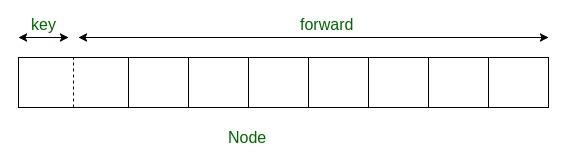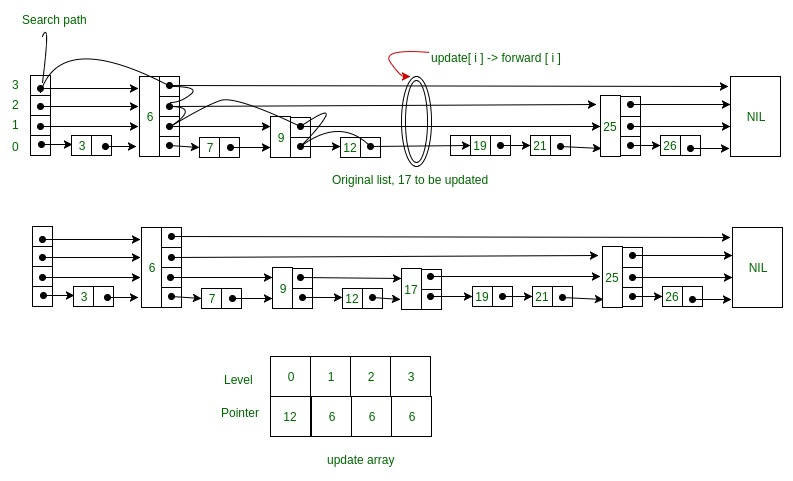# Skip List | Set 2 (Insertion)

• Difficulty Level : Hard
• Last Updated : 16 Dec, 2019

We have already discussed the idea of Skip list and how they work in Skip List | Set 1 (Introduction). In this article, we will be discussing how to insert an element in Skip list.

Deciding nodes level

Attention reader! Don’t stop learning now. Get hold of all the important DSA concepts with the DSA Self Paced Course at a student-friendly price and become industry ready.  To complete your preparation from learning a language to DS Algo and many more,  please refer Complete Interview Preparation Course.

In case you wish to attend live classes with experts, please refer DSA Live Classes for Working Professionals and Competitive Programming Live for Students.

Each element in the list is represented by a node, the level of the node is chosen randomly while insertion in the list. Level does not depend on the number of elements in the node. The level for node is decided by the following algorithm –

```randomLevel()
lvl := 1
//random() that returns a random value in [0...1)
while random() < p and lvl < MaxLevel do
lvl := lvl + 1
return lvl
```

MaxLevel is the upper bound on number of levels in the skip list. It can be determined as –. Above algorithm assure that random level will never be greater than MaxLevel. Here p is the fraction of the nodes with level i pointers also having level i+1 pointers and N is the number of nodes in the list.

Node Structure

Each node carries a key and a forward array carrying pointers to nodes of a different level. A level i node carries i forward pointers indexed through 0 to i.Insertion in Skip List

We will start from highest level in the list and compare key of next node of the current node with the key to be inserted. Basic idea is If –

1. Key of next node is less than key to be inserted then we keep on moving forward on the same level
2. Key of next node is greater than the key to be inserted then we store the pointer to current node i at update[i] and move one level down and continue our search.

At the level 0, we will definitely find a position to insert given key. Following is the pseudo code for the insertion algorithm –

```Insert(list, searchKey)
local update[0...MaxLevel+1]
for i := list -> level downto 0 do
while x -> forward[i] -> key  forward[i]
update[i] := x
x := x -> forward
lvl := randomLevel()
if lvl > list -> level then
for i := list -> level + 1 to lvl do
list -> level := lvl
x := makeNode(lvl, searchKey, value)
for i := 0 to level do
x -> forward[i] := update[i] -> forward[i]
update[i] -> forward[i] := x
```

Here update[i] holds the pointer to node at level i from which we moved down to level i-1 and pointer of node left to insertion position at level 0. Consider this example where we want to insert key 17 –Following is the code for insertion of key in Skip list –

## C++

 `// C++ code for inserting element in skip list`` ` `#include ``using` `namespace` `std;`` ` `// Class to implement node``class` `Node``{``public``:``    ``int` `key;`` ` `    ``// Array to hold pointers to node of different level ``    ``Node **forward;``    ``Node(``int``, ``int``);``};`` ` `Node::Node(``int` `key, ``int` `level)``{``    ``this``->key = key;`` ` `    ``// Allocate memory to forward ``    ``forward = ``new` `Node*[level+1];`` ` `    ``// Fill forward array with 0(NULL)``    ``memset``(forward, 0, ``sizeof``(Node*)*(level+1));``};`` ` `// Class for Skip list``class` `SkipList``{``    ``// Maximum level for this skip list``    ``int` `MAXLVL;`` ` `    ``// P is the fraction of the nodes with level ``    ``// i pointers also having level i+1 pointers``    ``float` `P;`` ` `    ``// current level of skip list``    ``int` `level;`` ` `    ``// pointer to header node``    ``Node *header;``public``:``    ``SkipList(``int``, ``float``);``    ``int` `randomLevel();``    ``Node* createNode(``int``, ``int``);``    ``void` `insertElement(``int``);``    ``void` `displayList();``};`` ` `SkipList::SkipList(``int` `MAXLVL, ``float` `P)``{``    ``this``->MAXLVL = MAXLVL;``    ``this``->P = P;``    ``level = 0;`` ` `    ``// create header node and initialize key to -1``    ``header = ``new` `Node(-1, MAXLVL);``};`` ` `// create random level for node``int` `SkipList::randomLevel()``{``    ``float` `r = (``float``)``rand``()/RAND_MAX;``    ``int` `lvl = 0;``    ``while` `(r < P && lvl < MAXLVL)``    ``{``        ``lvl++;``        ``r = (``float``)``rand``()/RAND_MAX;``    ``}``    ``return` `lvl;``};`` ` `// create new node``Node* SkipList::createNode(``int` `key, ``int` `level)``{``    ``Node *n = ``new` `Node(key, level);``    ``return` `n;``};`` ` `// Insert given key in skip list``void` `SkipList::insertElement(``int` `key)``{``    ``Node *current = header;`` ` `    ``// create update array and initialize it``    ``Node *update[MAXLVL+1];``    ``memset``(update, 0, ``sizeof``(Node*)*(MAXLVL+1));`` ` `    ``/*    start from highest level of skip list``        ``move the current pointer forward while key ``        ``is greater than key of node next to current``        ``Otherwise inserted current in update and ``        ``move one level down and continue search``    ``*/``    ``for` `(``int` `i = level; i >= 0; i--)``    ``{``        ``while` `(current->forward[i] != NULL &&``              ``current->forward[i]->key < key)``            ``current = current->forward[i];``        ``update[i] = current;``    ``}`` ` `    ``/* reached level 0 and forward pointer to ``       ``right, which is desired position to ``       ``insert key. ``    ``*/``    ``current = current->forward;`` ` `    ``/* if current is NULL that means we have reached``       ``to end of the level or current's key is not equal``       ``to key to insert that means we have to insert``       ``node between update and current node */``    ``if` `(current == NULL || current->key != key)``    ``{``        ``// Generate a random level for node``        ``int` `rlevel = randomLevel();`` ` `        ``// If random level is greater than list's current``        ``// level (node with highest level inserted in ``        ``// list so far), initialize update value with pointer``        ``// to header for further use``        ``if` `(rlevel > level)``        ``{``            ``for` `(``int` `i=level+1;iforward[i] = update[i]->forward[i];``            ``update[i]->forward[i] = n;``        ``}``        ``cout << ``"Successfully Inserted key "` `<< key << ``"\n"``;``    ``}``};`` ` `// Display skip list level wise``void` `SkipList::displayList()``{``    ``cout<<``"\n*****Skip List*****"``<<``"\n"``;``    ``for` `(``int` `i=0;i<=level;i++)``    ``{``        ``Node *node = header->forward[i];``        ``cout << ``"Level "` `<< i << ``": "``;``        ``while` `(node != NULL)``        ``{``            ``cout << node->key<<``" "``;``            ``node = node->forward[i];``        ``}``        ``cout << ``"\n"``;``    ``}``};`` ` `// Driver to test above code``int` `main()``{``    ``// Seed random number generator``    ``srand``((unsigned)``time``(0));`` ` `    ``// create SkipList object with MAXLVL and P ``    ``SkipList lst(3, 0.5);`` ` `    ``lst.insertElement(3);``    ``lst.insertElement(6);``    ``lst.insertElement(7);``    ``lst.insertElement(9);``    ``lst.insertElement(12);``    ``lst.insertElement(19);``    ``lst.insertElement(17);``    ``lst.insertElement(26);``    ``lst.insertElement(21);``    ``lst.insertElement(25);``    ``lst.displayList();``}`

## Python

 `# Python3 code for inserting element in skip list`` ` `import` `random`` ` `class` `Node(``object``):``    ``'''``    ``Class to implement node``    ``'''``    ``def` `__init__(``self``, key, level):``        ``self``.key ``=` `key`` ` `        ``# list to hold references to node of different level ``        ``self``.forward ``=` `[``None``]``*``(level``+``1``)`` ` `class` `SkipList(``object``):``    ``'''``    ``Class for Skip list``    ``'''``    ``def` `__init__(``self``, max_lvl, P):``        ``# Maximum level for this skip list``        ``self``.MAXLVL ``=` `max_lvl`` ` `        ``# P is the fraction of the nodes with level ``        ``# i references also having level i+1 references``        ``self``.P ``=` `P`` ` `        ``# create header node and initialize key to -1``        ``self``.header ``=` `self``.createNode(``self``.MAXLVL, ``-``1``)`` ` `        ``# current level of skip list``        ``self``.level ``=` `0``     ` `    ``# create  new node``    ``def` `createNode(``self``, lvl, key):``        ``n ``=` `Node(key, lvl)``        ``return` `n``     ` `    ``# create random level for node``    ``def` `randomLevel(``self``):``        ``lvl ``=` `0``        ``while` `random.random()<``self``.P ``and` `\``              ``lvl<``self``.MAXLVL:lvl ``+``=` `1``        ``return` `lvl`` ` `    ``# insert given key in skip list``    ``def` `insertElement(``self``, key):``        ``# create update array and initialize it``        ``update ``=` `[``None``]``*``(``self``.MAXLVL``+``1``)``        ``current ``=` `self``.header`` ` `        ``'''``        ``start from highest level of skip list``        ``move the current reference forward while key ``        ``is greater than key of node next to current``        ``Otherwise inserted current in update and ``        ``move one level down and continue search``        ``'''``        ``for` `i ``in` `range``(``self``.level, ``-``1``, ``-``1``):``            ``while` `current.forward[i] ``and` `\``                  ``current.forward[i].key < key:``                ``current ``=` `current.forward[i]``            ``update[i] ``=` `current`` ` `        ``''' ``        ``reached level 0 and forward reference to ``        ``right, which is desired position to ``        ``insert key.``        ``'''` `        ``current ``=` `current.forward[``0``]`` ` `        ``'''``        ``if current is NULL that means we have reached``           ``to end of the level or current's key is not equal``           ``to key to insert that means we have to insert``           ``node between update and current node``       ``'''``        ``if` `current ``=``=` `None` `or` `current.key !``=` `key:``            ``# Generate a random level for node``            ``rlevel ``=` `self``.randomLevel()`` ` `            ``'''``            ``If random level is greater than list's current``            ``level (node with highest level inserted in ``            ``list so far), initialize update value with reference``            ``to header for further use``            ``'''``            ``if` `rlevel > ``self``.level:``                ``for` `i ``in` `range``(``self``.level``+``1``, rlevel``+``1``):``                    ``update[i] ``=` `self``.header``                ``self``.level ``=` `rlevel`` ` `            ``# create new node with random level generated``            ``n ``=` `self``.createNode(rlevel, key)`` ` `            ``# insert node by rearranging references ``            ``for` `i ``in` `range``(rlevel``+``1``):``                ``n.forward[i] ``=` `update[i].forward[i]``                ``update[i].forward[i] ``=` `n`` ` `            ``print``(``"Successfully inserted key {}"``.``format``(key))`` ` `    ``# Display skip list level wise``    ``def` `displayList(``self``):``        ``print``(``"\n*****Skip List******"``)``        ``head ``=` `self``.header``        ``for` `lvl ``in` `range``(``self``.level``+``1``):``            ``print``(``"Level {}: "``.``format``(lvl), end``=``" "``)``            ``node ``=` `head.forward[lvl]``            ``while``(node !``=` `None``):``                ``print``(node.key, end``=``" "``)``                ``node ``=` `node.forward[lvl]``            ``print``("")`` ` `# Driver to test above code``def` `main():``    ``lst ``=` `SkipList(``3``, ``0.5``)``    ``lst.insertElement(``3``)``    ``lst.insertElement(``6``)``    ``lst.insertElement(``7``)``    ``lst.insertElement(``9``)``    ``lst.insertElement(``12``)``    ``lst.insertElement(``19``)``    ``lst.insertElement(``17``)``    ``lst.insertElement(``26``)``    ``lst.insertElement(``21``)``    ``lst.insertElement(``25``)``    ``lst.displayList()`` ` `main()`

Output:
```Successfully Inserted key 3
Successfully Inserted key 6
Successfully Inserted key 7
Successfully Inserted key 9
Successfully Inserted key 12
Successfully Inserted key 19
Successfully Inserted key 17
Successfully Inserted key 26
Successfully Inserted key 21
Successfully Inserted key 25

*****Skip List*****
Level 0: 3 6 7 9 12 17 19 21 25 26
Level 1: 3 6 12 17 25
Level 2: 6 12 17 25
Level 3: 12 17 25
```

Note: The level of nodes is decided randomly, so output may differ.
Time complexity (Average):Time complexity (Worst):In next article we will discuss searching and deletion in Skip List.
References

This article is contributed by Atul Kumar. If you like GeeksforGeeks and would like to contribute, you can also write an article using contribute.geeksforgeeks.org or mail your article to contribute@geeksforgeeks.org. See your article appearing on the GeeksforGeeks main page and help other Geeks.